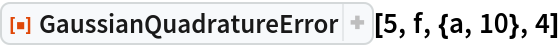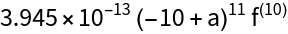Function Repository Resource:

Find the error in the Gaussian quadrature approximation of a function’s integral

Contributed by: Paul Abbott (additional contributions by Wolfram Research staff)
 ResourceFunction["GaussianQuadratureError"][n,f,a,b] gives the leading term in the error of the elementary n-point Gaussian quadrature formula for the function f on an interval from a to b. ResourceFunction["GaussianQuadratureError"][n,f,a,b,prec] attempts to give a result with prec digits of precision.

## Details and Options

The function f may be a pure function or a named function defined using Set or SetDelayed.
The error is given as a function of n, a and b multiplied by an mth derivative of the function f. The size of the error is bounded by the maximum of this expression over the interval from a to b.

## Examples

### Basic Examples (2)

The GaussianQuadratureError depends on the 10th integral at an unknown abscissa:

 In:=Out=Use the specified precision:

 In:=Out=## Version History

• 2.0.0 – 06 November 2020
• 1.0.0 – 20 November 2019